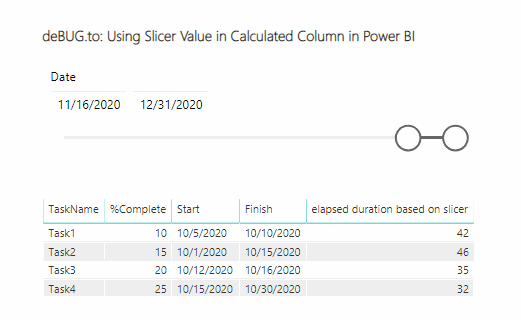# Power BI calculated column based on Slicer

1 like 0 dislike
6.9k views
in Power BI

How I can use slicer value in calculated column in power bi to calculate and show data automatically in a table when the slicer value changed?

I need to use the selected slicer value in a calculated column to calculate the elapsed days in my table based on the selected date from slicer.

I have used power bi measure based on slicer to get the value from a measure then I used this measure in the caulated column but the calculated column in table is not changed when I change the date in slicer!

1 like 0 dislike
by 71 141 308
selected by

## Using Slicer Value in Calculated Column in Power BI

First of all, you should be aware of the Calculated Column has more impact on the Memory than the measure, also it will not be recalculated until the data model is refreshed.

So, you should use a measure instead of the calculated column if you would like to change the calculation in a table when the slicer value changed.

### Calculate elapsed days in a table based on slicer in Power

To pass slicer value in a table and calculate the value when the slicer value changed you should do the following:

Steps

1. Get the Slicer value in a measure as mentioned at power bi measure based on slicer.
2. In your case, you Create a new measure to calculate the date difference between the date slicer value and the date field in your table as below:

`````` elapsed duration based on slicer = DATEDIFF(FIRSTDATE('Tasks'[Start]),'Calendar'[Slicer Selection],DAY)
``````

OutputNote: you should use FIRSTDATE() function to calculated the elapsed value per each row.by